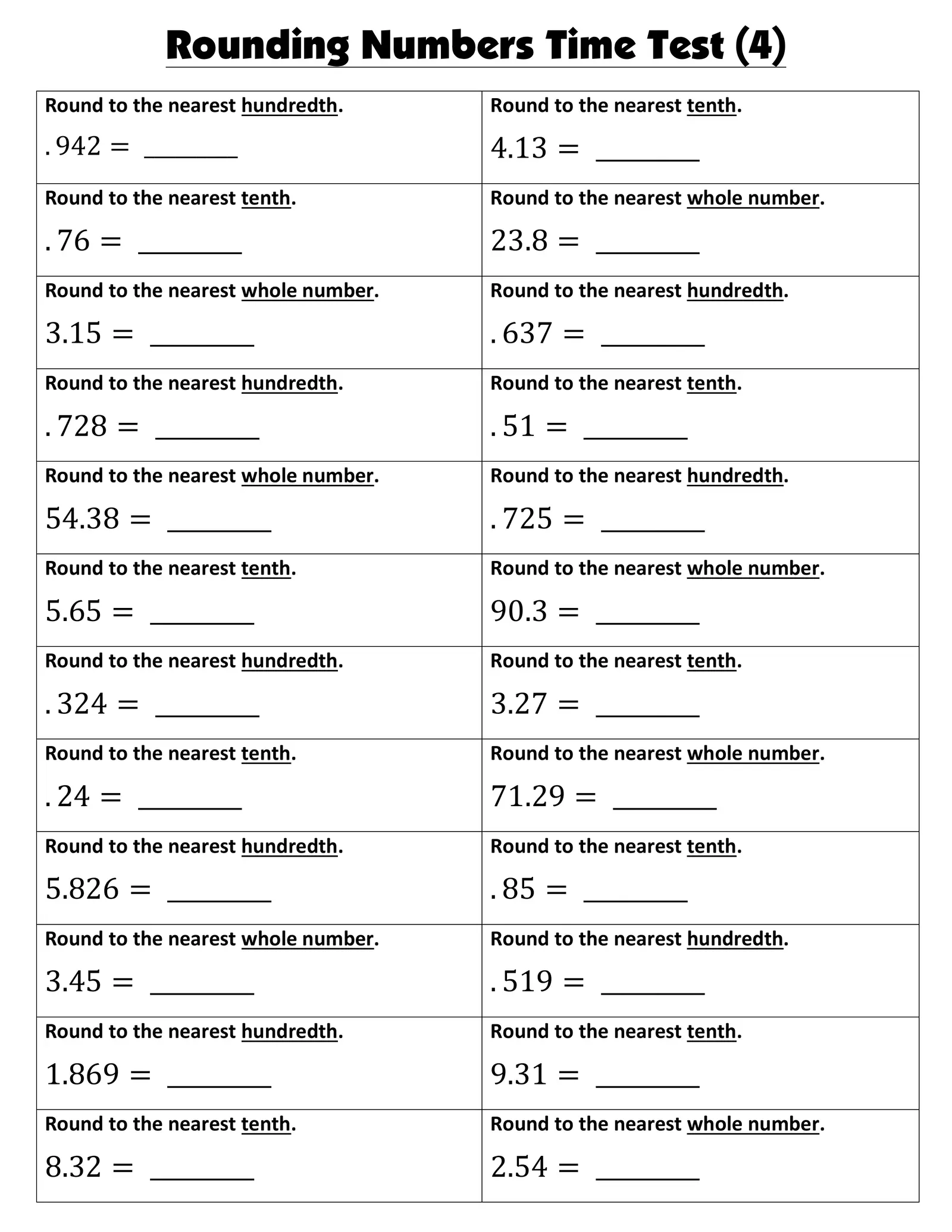# ## Rounding Numbers Time Test (4) ##

Created

Rounding Numbers Time Test (4) Round to the nearest hundredth. Round to the nearest tenth. . 942 = _________ 4.13 = _________ Round to the nearest tenth. Round to the nearest whole number. . 76 = _________ 23.8 = _________ Round to the nearest whole number. Round to the nearest hundredth. 3.15 = _________ . 637 = _________ Round to the nearest hundredth. Round to the nearest tenth. . 728 = _________ . 51 = _________ Round to the nearest whole number. Round to the nearest hundredth. 54.38 = _________ . 725 = _________ Round to the nearest tenth. Round to the nearest whole number. 5.65 = _________ 90.3 = _________ Round to the nearest hundredth. Round to the nearest tenth. . 324 = _________ 3.27 = _________ Round to the nearest tenth. Round to the nearest whole number. . 24 = _________ 71.29 = _________ Round to the nearest hundredth. Round to the nearest tenth. 5.826 = _________ . 85 = _________ Round to the nearest whole number. Round to the nearest hundredth. 3.45 = _________ . 519 = _________ Round to the nearest hundredth. Round to the nearest tenth. 1.869 = _________ 9.31 = _________ Round to the nearest tenth. Round to the nearest whole number. 8.32 = _________ 2.54 = _________